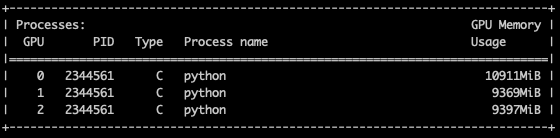# Extremely unequal load balance across multiple gpus

Hi all,

I have a model based on Bert (by using HuggingFace’s implementation) and MLP. I am trying to train it by using 3 gpus I have.
Unfortunately, my code uses 10 Gb of available 11 GB gpu memory in the first gpu and only 500 megabytes in the second and third GPUs. Here is the screenshot of it:Here is the model and the code I use to initialize and train the model:

``````class MODEL_BERT_SUM_MLP(nn.Module):
def __init__(self, input_size, hidden_size, dropout, output_size=2):
super(MODEL_BERT_SUM_MLP, self).__init__()
self.input_size   = input_size
self.hidden_size  = hidden_size
self.output_size  = output_size
self.bert         = BertModel.from_pretrained('bert-base-cased')
self.outlin       = nn.Linear(300,self.output_size)
self.outlin1       = nn.Linear(self.input_size,300)
self.activation   = nn.ReLU()
self.dropout      =nn.Dropout(p=dropout)
self.loss_func    = nn.CrossEntropyLoss()

def forward(self,input_ids=None, attention_mask=None, token_type_ids=None, labels=None):
a,pooled_output = self.bert(input_ids,
token_type_ids=token_type_ids)
# Here I am summing over the batch dimension. This is not a mistake, I need to do this,
# is it safe to do that on Multi-gpu setting ?
out = torch.sum(pooled_output,dim=0,keepdim=True)
out = self.dropout(self.activation(self.outlin1(out)))
out = self.outlin(out)
lss = self.loss_func(out,labels)
return (out,lss)
``````
``````device = torch.device("cuda" if torch.cuda.is_available() else "cpu")
model = MODEL_BERT_SUM_MLP(args.embed_size,args.hidden_size,args.dropout).to(device)
model = torch.nn.DataParallel(model)
optimizer = AdamW(model.parameters(),lr=args.lr, eps=1e-8)
scheduler = get_linear_schedule_with_warmup(optimizer,
num_warmup_steps = 0,
num_training_steps = total_steps)
for step,batch in enumerate(corpus.iter_batches(batch_size=args.bs,split_name='train',shuffle=True)):

batch_input_ids=[torch.tensor(d_input_ids).to(device) for d_input_ids in batch]
batch_segment_ids = [torch.tensor(d_segment_ids).to(device) for d_segment_ids in batch]
batch_labels = torch.tensor(batch).to(device)

logits,loss = model(input_ids=batch_input_ids,
token_type_ids=batch_segment_ids,
labels = batch_labels)
loss = loss.mean()
``````

How can I solve this problem ? Is there anyone to help me on this ? Thanks.

EDIT

Note that, Unlike here, I am not using a DataLoader inhereted from (Dataset) class of PyTorch. Would it be a problem ?

EDIT2
Also note that, in my forward function, I am summing the elements in a batch:
`out = torch.sum(pooled_output,dim=0,keepdim=True)`

I did it because each row in my batch is a different section of a document. I get a representation for each section by using bert independently and then I sum them all to get a single representation for the document. Is this why PyTorch could not divide the batch into gpus?

EDIT3
When I modify the constructor of the model as below ( and remove the line ` model = torch.nn.DataParallel(model)` ), I observe a better memory balance across GPUs. However, I am now even not sure if these two codes are identical or not.

``````class MODEL_BERT_SUM_MLP(nn.Module):
def __init__(self, input_size, hidden_size, dropout, output_size=2):
super(MODEL_BERT_SUM_MLP, self).__init__()
self.input_size   = input_size
self.hidden_size  = hidden_size
self.output_size  = output_size
self.bert         = BertModel.from_pretrained('bert-base-cased')
# THIS LINE IS NEW, now I am only making bert component multi-gpu
self.bert          = torch.nn.DataParallel(self.bert)
self.outlin       = nn.Linear(300,self.output_size)
self.outlin1       = nn.Linear(self.input_size,300)
self.activation   = nn.ReLU()
self.dropout      =nn.Dropout(p=dropout)
self.loss_func    = nn.CrossEntropyLoss()
``````Hi @ptrblck, could you, if possible, help me on this issue ?

It seems that wrapping the complete model into `nn.DataParallel` uses less memory on GPU1 and 2 than wrapping just a single part of the model?

The imbalance is expected, as the default device will be used to gather the input data, loss etc. as explained here.
What batch size are you using in both approaches?

1 Like